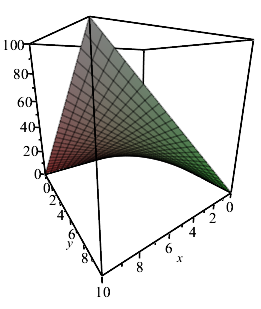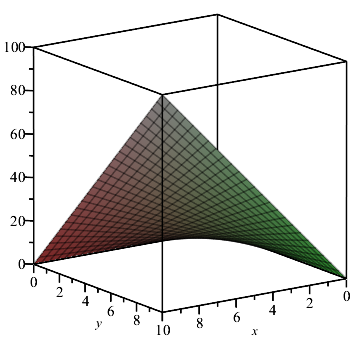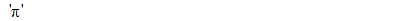## How to create a sequence which depends on summatio...

Each term of the following sequence is derived using the sum of all preceding terms following a simple algorithm. I have tried to code it but without success (keep getting error message "too many levels of recursion").

Definition is as follows:

a(0)=0, a(1)=1;  for n>=1,

a(n+2) = n + Sum{k=1..n+1} a(k).

it starts: 0,1,2,5,11,23,47,95,191,767,1536,3071,6143...

(Looks like every prime term is of the form 3*2^m-1).

Cann anyone suggest a solution?

Thanks,

David.

## Difference between seq and \$ that explains a diffe...

Hi,

While computing a simple sequence of numbers, I remarked a small difference between the result obtained with \$ and with seq.

```Digits:=20:
u:=proc(n) evalf(((n+1)/n)^(n+1)) end proc:
a:=[u(n)\$n=1..10]:
b:=[seq(u(n),n=1..10)]:
a-b;```

The result is:

```[0., 0., -4.*10^(-19), 0., 0., 6.*10^(-19), -9.*10^(-19), 0., -3.*10^(-19), 0.]
```

I have no idea why there are nonzero values in the output. Does anyone have an explanation?

It seems I get the correct values if I quote the expression before \$:

`a:=['u(n)'\$n=1..10]:`

However, it's not clear to me why this makes a difference in evalf.

## Is there any simplified code for classification ...

As the title says， I'd like to  divide Non-isomorphism  graphs of order 7   into 6 parts according  diameter of graph.

I write following codes:

restart:
with(GraphTheory):
for i from 1 to 7 do
D||i:=[]:
od:

prc:=NonIsomorphicGraphs(7,output=iterator,outputform=graph,restrictto = connected ):
graphmark := prc():
while graphmark <> FAIL  do
if Diameter(graphmark) = 1 then
D1:=[op(D1),graphmark];
elif Diameter(graphmark) = 2 then
D2:=[op(D2),graphmark];
elif Diameter(graphmark) = 3 then
D3:=[op(D3),graphmark];
elif Diameter(graphmark) = 4 then
D4:=[op(D4),graphmark];
elif Diameter(graphmark) = 5 then
D5:=[op(D5),graphmark];
elif Diameter(graphmark) = 6 then
D6:=[op(D6),graphmark];
fi:
graphmark:=prc():
end do:

But I think  conditional statements  if.. elif are used to many  in  above maple  codes,  it is not concise.

How to write better?

## Bug - projection will not work with orientation...

Projection will not work with orientation.

plot3d(x*y, x = 0 .. 10, y = 0 .. 10, projection = 0.2)plot3d(x*y, x = 0 .. 10, y = 0 .. 10, projection = 0.2, orientation = [55, 75, 0])## Weird numerical evaluation...

After setting:

Digits:=20;

result of:

evalhf(9.3653976306373904636884810627704*10^(-315));

is:

-9.0813464423699167205*10^(-315)

This looks nonsesnse. What is going on?

## transcendental equation...

Hello everyone I am trying to solve the transcendental equation by using the regular false position method cannot succeed. is their nay can solve it form me...

F := ((-288*(lambda + (2*k)/3 - 2/3)*sqrt(2)*lambda^2*arctan(sqrt(4*lambda/(1 - k) - 2)*sqrt(2)/2)/(1 - k)^2 - 144*(lambda + (2*k)/3 - 2/3)*Pi*lambda^2*sqrt(2)/(1 - k)^2 - 36*((lambda + (2*k)/3 - 2/3)*(4*lambda/(1 - k) - 2) + (10*lambda)/3 + (4*k)/3 - 4/3)*sqrt(4*lambda/(1 - k) - 2))*(1 - k)^2)/(256*lambda^2)

## how to show which region of the plan points belong...

how to show which region of the plan belongs to the argument points between 0 and Pi/2 and the module points between 0 and 2 ?

## How to open .mm file in Maple...

Does anyone know how to open .mm file in Maple, as well as using the program or function in .mm file in Maple? Many thanks.

## The Order Term Function simplification O(x^n)/x^(n...

Hello colleagues,

I would like to get a suggestion on how to utilize an existing `simplify` function or to define an operator, which allows the following simplification:— Alexander

## combinat:-subset with a chosen number of elements?...

Hello

I need to test a huge amount of subsets and for doing that I was thinking of using combinat:-subset, since it avoids allocating memory for all subsets.  The problem with subset for my specific application is that it generates sets with all possible sizes and I just need subsets with a chosen number of elements.  Can that be done in maple?

Many thanks

Ed

## Can points in a graph each be an active (click) l...

I have a plot of points in a graphical plot on the domain (x,y).  At each point xi (i=1..N) , there is a numerical value yi = S(xi) from which I plot a series of points (xi, S(xi)) in the (x,y) domain.

Each point (xi,yi) represents a transient plot of two variables u(x,t) and v(x,t) in a plot file.  Is it possible to define a graph of the points (xi, S(xi)), such that clicking on any such point in the graph opens up a graphical file which is parameterised by (xi, S(xi))?

Would welcome help on this...

Melvin

## When running a for do cycle the first two solution...

Hi,

when running a for do cycle the first two solutions are exact the same numbers, which is not correct. Can someone plesa help me fix this problem?

Thanks a lot.

 > restart
 > tmin:=10: #°C
 > tmax:=70: #°C
 > Tmin:=tmin+273.15:
 > Tmax:=tmax+273.15:
 > T:='T':
 > i:=0:
 >
 > for T from Tmin by 2 to Tmax+1.25 do i:=i+1: a12:=2305.28444347652 - 9.14490843016421*T + 0.00680052257590234*T^2: a21:=-6665.24838284836 + 46.0897018087247*T - 0.0694991633494123*T^2: alfa:=0.3: x2:=1-x1: lng1:=x2^2*(tau21*(G21/(x1+x2*G21))^2+tau12*(G12/((x2+x1*G12)^2))): lng2:=x1^2*(tau12*(G12/(x2+x1*G12))^2+tau21*(G21/((x1+x2*G21)^2))): G12:=exp(-alfa*tau12): G21:=exp(-alfa*tau21): tau12:=a12/T: tau21:=a21/T: lnga1:=subs(x1=xa1,lng1): lngb1:=subs(x1=xb1,lng1): lnga2:=subs(x1=xa1,lng2): lngb2:=subs(x1=xb1,lng2): r1:=lnga1+ln(xa1)=lngb1+ln(xb1): r2:=lnga2+ln(1-xa1)=lngb2+ln(1-xb1): r:=fsolve({r1,r2},{xa1=0..0.22,xb1=0.22..1}): assign(r): xA1[i]:=xa1: xB1[i]:=xb1: print(i,T,xA1[i],xB1[i]); unassign('r1','r2','r','xa1','xb1'): od:(1)
 >

lle.mw

## Prevent procedure from accessing (global) workshee...

Dear MaplePrimes community,

I am currently struggling to use the simplify-command in my toolbox for symbolic generation of robot dynamics.

I created a procedure which calls the simplify command with some extra checks. There are symbolic expressions in the term to be simplified that also occur in the workspace of the worksheet calling the procedure.

The simplify command (called from within the procedure) shall regard them as symbolic expressions unaware of the content of the variable in the workspace. Nevertheless, the simplifications are performed with the global variables, even though they are declared local to the procedure.

Minimal example: The variable "l1" stands for "length 1" in a mechanic problem as well as "length of the expression"

```restart:
with(LinearAlgebra): with(ArrayTools): with(codegen): with(CodeGeneration): with(StringTools):
T := m2*(l1+l2)*(qD2)^2: # dummy-expression for kinetic energy
l1 := length(T);
T_simpl := simplify2(T):
l2 := length(T_simpl);
```

Code for the file proc_simplify2:

```simplify2 := proc (Term)
# In some cases the term gets longer after the simplification. Then discard.
# Give the local procedure variable a long name. They must not be identical in Term.
local simplify_tmpvar_c1, simplify_tmpvar_c1sum, simplify_tmpvar_c2, \
simplify_tmpvar_c2sum, simplify_tmpvar_nms, simplify_tmpvar_k, Term2:
# Assume all contents of the Term to be local variables to this procedure
# Otherwise, variables like "l1" can be overwritten by occurences in the calling worksheet.
simplify_tmpvar_nms:=convert(indets(Term,name),list): # get list of symbols
for simplify_tmpvar_k from 1 to ColumnDimension(simplify_tmpvar_nms) do
if simplify_tmpvar_nms[simplify_tmpvar_k] = Pi or simplify_tmpvar_nms[simplify_tmpvar_k] = 0 then
next: # 0 and Pi can not be variables
end if:
eval(sprintf("local %s;", String(simplify_tmpvar_nms[simplify_tmpvar_k]))); # assume local
end do:

# Get computational effort before simplification
simplify_tmpvar_c1sum := diff(simplify_tmpvar_c1,functions)+ \
diff(simplify_tmpvar_c1,multiplications)+\
diff(simplify_tmpvar_c1,divisions):
# Perform simplification. Attention: tries to use global variables to simplify the expression (see above)
Term2 := simplify(Term):
# Get effort after simplification
simplify_tmpvar_c2sum := diff(simplify_tmpvar_c2,functions)+\
diff(simplify_tmpvar_c2,multiplications)+\
diff(simplify_tmpvar_c2,divisions):
if simplify_tmpvar_c2sum > simplify_tmpvar_c1sum then
# The simplification was not successful. Take old version.
Term2 := Term:
end if:
return Term2:
end proc:
```

I would be glad for suggestions how to define the symbolic expressions in Term as local to the procedure or perhaps a better way to perform simplifications. As my program extends over several worksheets and the user may define an input file, I would not like to have to control all names of temporary variables that may occur at some point in the project. Until I tried to use the simplify-command, there was no problem regarding variable names. Is it perhaps possible that the variables are now only declared local in the for loop and not in the procedure?

## basic question on evaluation delay...

Why

`evalf(evalf(''Pi''))`

ProducesBut

```evalf(''Pi''):
evalf(%)```

Produces

3.141592654